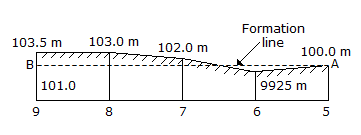# Civil Engineering - Estimating and Costing - Discussion

### Discussion :: Estimating and Costing - Section 1 (Q.No.35)

35.

The reduced levels of points, 30 metres apart along the longitudinal section of a road portion between chainages 5 and 9 are shown in the given figure. If there is a uniform up-gradient of the road 120 in 1, the chainage of the point with no filling or cutting is[A]. (6 + 15) chains [B]. (6 + 12) chains [C]. (6 + 18) chains [D]. None of these.

Explanation:

No answer description available for this question.

 Saikumar said: (Sep 17, 2015) How it is calculated?

 Prabhakar said: (Sep 27, 2015) How it is taken?

 Gance said: (Dec 25, 2015) 1 in 120. So for 30 m there is rise of 0.25 m. R.L of formation level at 6 chain = 100+0.25 = 100.25. Now apply the similar triangles as 1/x = 1.5/(30-X). Where, 1 = 100.25-99.25. x = 12. So the answer B.

 Ashik said: (Aug 11, 2016) Can you explain it? Please.

 Kamruz said: (Jan 6, 2017) I think it is A.

 Asmaul Husna said: (May 9, 2017) @Gance. Where is 1.5 from?

 Karthick said: (Jun 24, 2017) 6+ 12 chain how?

 Vishnu said: (Jul 11, 2017) Can anyone explain clearly?

 Chandra said: (Nov 28, 2017) How this will occurs?

 Maya said: (Dec 20, 2017) @6 difference b/w level is (100.25-99.25)=1. @7 RL=100.25+0.25=100.5. So difference= 102-100.5=1.5, let x be distance at no filling or cutting, distance b/w 7 and 6 is 30, By similar triangle, 1 -> x, 1.5 ->30-x.

 Liam Payne said: (Jan 10, 2018) The number of neutrons will be 6 so the total number becomes 6 + 6 is equal to 12.

 Mainak said: (Jun 6, 2018) 99.25+(2.75/30)*x = 100+(x+30)/120. By solving it x=12m from chainage 6 towards ch. 7, so answer will be (6+12).

 Abhishek said: (Dec 13, 2018) It is (6+8) chains.

 Debika said: (Jun 4, 2020) Please, explain this.